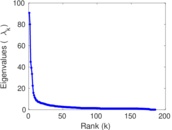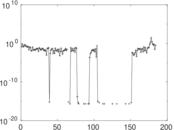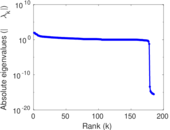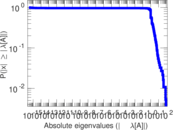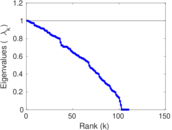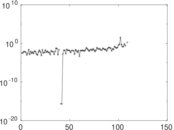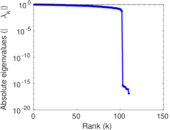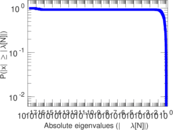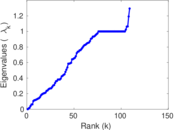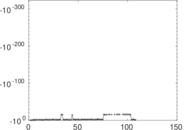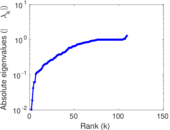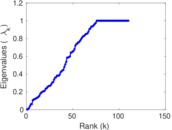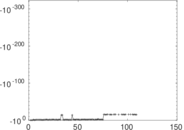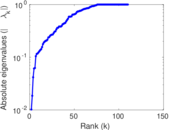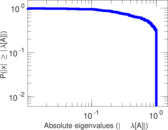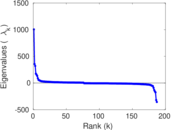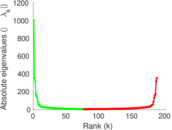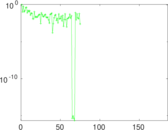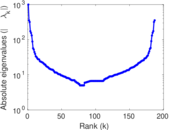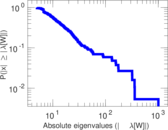# Wiktionary edits (ha)

This is the bipartite edit network of the Hausa Wiktionary. It contains users and pages from the Hausa Wiktionary, connected by edit events. Each edge represents an edit. The dataset includes the timestamp of each edit.

 Code `mha` Internal name `edit-hawiktionary` Name Wiktionary edits (ha) Data source http://dumps.wikimedia.org/ AvailabilityDataset is available for download Consistency checkDataset passed all tests Category Authorship network Dataset timestamp 2017-10-20 Node meaning User, article Edge meaning Edit Network formatBipartite, undirected Edge typeUnweighted, multiple edges Temporal dataEdges are annotated with timestamps

## Statistics

 Size n = 1,017 Left size n1 = 187 Right size n2 = 830 Volume m = 2,563 Unique edge count m̿ = 1,523 Wedge count s = 71,036 Claw count z = 3,796,263 Cross count x = 173,912,192 Square count q = 24,447 4-Tour count T4 = 483,074 Maximum degree dmax = 533 Maximum left degree d1max = 533 Maximum right degree d2max = 118 Average degree d = 5.040 31 Average left degree d1 = 13.705 9 Average right degree d2 = 3.087 95 Fill p = 0.009 812 51 Average edge multiplicity m̃ = 1.682 86 Size of LCC N = 743 Diameter δ = 15 50-Percentile effective diameter δ0.5 = 4.957 04 90-Percentile effective diameter δ0.9 = 7.843 88 Median distance δM = 5 Mean distance δm = 5.210 32 Gini coefficient G = 0.724 638 Balanced inequality ratio P = 0.210 496 Left balanced inequality ratio P1 = 0.153 336 Right balanced inequality ratio P2 = 0.269 996 Relative edge distribution entropy Her = 0.828 206 Power law exponent γ = 3.207 42 Tail power law exponent γt = 2.151 00 Tail power law exponent with p γ3 = 2.151 00 p-value p = 0.000 00 Left tail power law exponent with p γ3,1 = 1.751 00 Left p-value p1 = 0.762 000 Right tail power law exponent with p γ3,2 = 2.321 00 Right p-value p2 = 0.000 00 Degree assortativity ρ = −0.225 561 Degree assortativity p-value pρ = 5.080 00 × 10−19 Spectral norm α = 90.708 4 Algebraic connectivity a = 0.010 206 7 Spectral separation |λ1[A] / λ2[A]| = 1.134 55 Controllability C = 659 Relative controllability Cr = 0.649 261

## Plots

### Fruchterman–Reingold graph drawing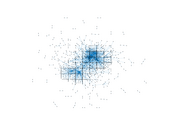### Degree distribution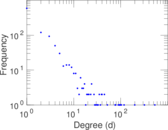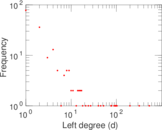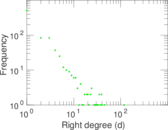### Cumulative degree distribution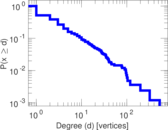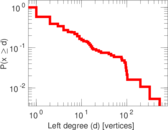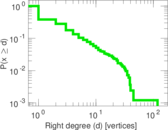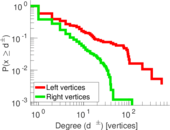### Lorenz curve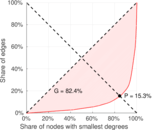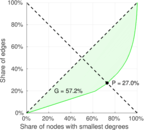### Spectral distribution of the adjacency matrix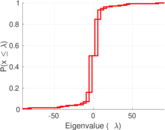### Spectral distribution of the normalized adjacency matrix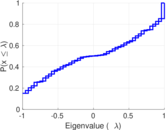### Spectral distribution of the Laplacian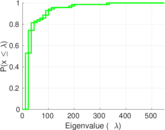### Spectral graph drawing based on the adjacency matrix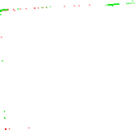### Spectral graph drawing based on the Laplacian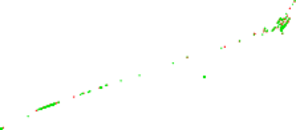### Spectral graph drawing based on the normalized adjacency matrix### Degree assortativity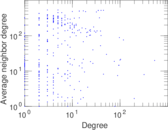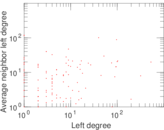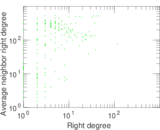### Zipf plot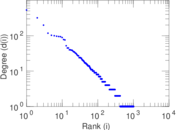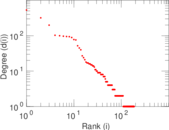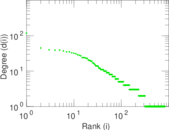### Hop distribution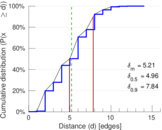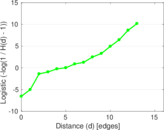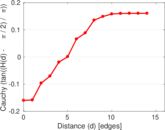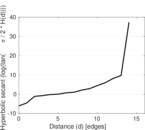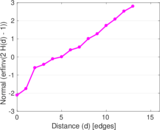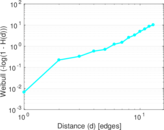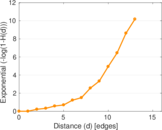### Double Laplacian graph drawing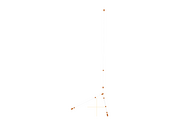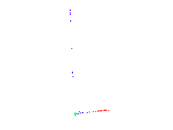### Delaunay graph drawing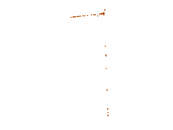### Edge weight/multiplicity distribution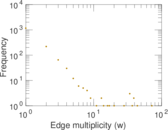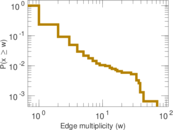### Temporal distribution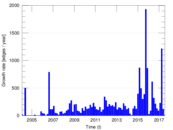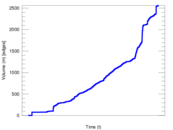### Temporal hop distribution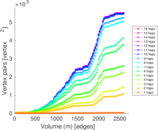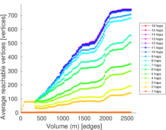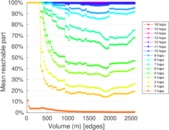### Diameter/density evolution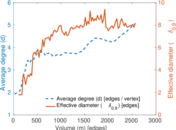### Matrix decompositions plots